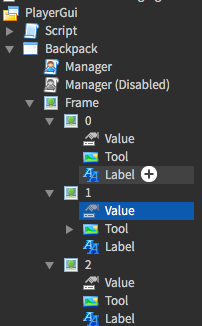# How would I make an inventory system?

So I’m making a game where you can build stuff, and you need wood, among other materials to get stuff. I’ve made the inventory system based on this tutorial: How to Make a Custom Backpack GUI | Roblox Scripting Tutorial - YouTube
I have already made the tool go inside the backpack when the player crafts an object, however I don’t want it to look like this:Currently, I am trying to make a string value that is in every slot, and will change depending on what is stored in that inventory slot. It also makes a integer value that stores how many of each it is storing.Then, whenever the player crafts something new, it checks whether that item is already there.
How would I accomplish this? The code is:

``````

game:GetService('StarterGui'):SetCoreGuiEnabled(Enum.CoreGuiType.Backpack, false)

--< variables >--
local uis = game:GetService("UserInputService")
local player = game.Players.LocalPlayer
local char = workspace:WaitForChild(player.Name)
local bp = player.Backpack
local hum = char:WaitForChild("Humanoid")
local frame = script.Parent.Frame
local template = frame.Template
local equipped = 0.2 -- icon transparencies
local unequipped = 0.7

local iconSize = template.Size
local iconBorder = {x = 15, y = 5} -- pixel space between icons

local inputKeys = { -- dictionary for effective referencing
["One"] = {txt = "1"},
["Two"] = {txt = "2"},
["Three"] = {txt = "3"},
["Four"] = {txt = "4"},
["Five"] = {txt = "5"},
["Six"] = {txt = "6"},
["Seven"] = {txt = "7"},
["Eight"] = {txt = "8"},
["Nine"] = {txt = "9"},
["Zero"] = {txt = "0"},
}

local inputOrder = { -- array for storing the order of the keys
inputKeys["One"],inputKeys["Two"],inputKeys["Three"],inputKeys["Four"],inputKeys["Five"],
inputKeys["Six"],inputKeys["Seven"],inputKeys["Eight"],inputKeys["Nine"],inputKeys["Zero"],
}

--< functions >--
function handleEquip(tool)
if tool then
if tool.Parent ~= char then
hum:EquipTool(tool)
else
hum:UnequipTools()
end
end
end

function create() -- creates all the icons at once (and will only run once)

local toShow = #inputOrder -- # operator can only be used with an array, not a dictionary
local totalX = (toShow*iconSize.X.Offset)+((toShow+1)*iconBorder.x)
local totalY = iconSize.Y.Offset + (2*iconBorder.y)

frame.Size = UDim2.new(0, totalX, 0, totalY)
frame.Position = UDim2.new(0.5, -(totalX/2), 1, -(totalY+(iconBorder.y*2)))
frame.Visible = true -- just in case!

for i = 1, #inputOrder do

local value = inputOrder[i]
local clone = template:Clone()
clone.Parent = frame
clone.Label.Text = value["txt"]
clone.Name = value["txt"]
clone.Visible = true
clone.Position = UDim2.new(0, (i-1)*(iconSize.X.Offset)+(iconBorder.x*i), 0, iconBorder.y)
clone.ImageTransparency = unequipped

local tool = value["tool"]
if tool then
clone.Tool.Image = tool.TextureId
local ToolSlotOccupant = Instance.new("StringValue", clone.Tool)
ToolSlotOccupant.Name = tool.Name
ToolSlotOccupant.Value = tool.Name
end

clone.Tool.MouseButton1Down:Connect(function() -- click icon to equip/unequip
for key, value in pairs(inputKeys) do
if value["txt"] == clone.Name then
handleEquip(value["tool"])
end
end
end)

end
template:Destroy()
end

function setup() -- sets up all the tools already in the backpack (and will only run once)
local tools = bp:GetChildren()
for i = 1, #tools do
if tools[i]:IsA("Tool") then -- does not assume that all objects in the backpack will be a tool (2.11.18)
for i = 1, #inputOrder do
local value = inputOrder[i]
if not value["tool"] then -- if the tool slot is free...
value["tool"] = tools[i]
break -- stop searching for a free slot
end
end
end
end
create()
end

for key, value in pairs(inputKeys) do
local tool = value["tool"]
local icon = frame:FindFirstChild(value["txt"])
if tool then
icon.Tool.Image = tool.TextureId
if tool.Parent == char then -- if the tool is equipped...
icon.ImageTransparency = equipped
else
icon.ImageTransparency = unequipped
end
else
icon.Tool.Image = ""
icon.ImageTransparency = unequipped
end
end
end

function onKeyPress(inputObject) -- press keys to equip/unequip
local key = inputObject.KeyCode.Name
local value = inputKeys[key]
if value and uis:GetFocusedTextBox() == nil then -- don't equip/unequip while typing in text box
handleEquip(value["tool"])
end
end

local new = true
--local ToolSlotOccupant = Instance.new("StringValue", clone.Tool)
--ToolSlotOccupant.Name = tool.Name
--ToolSlotOccupant.Value = tool.Name

for key, value in pairs(inputKeys) do
local tool = value["tool"]
if tool then
new = false
end
end
end

if new then
for i = 1, #inputOrder do
local tool = inputOrder[i]["tool"]
if not tool then -- if the tool slot is free...
break
end
end
end

end
end

function handleRemoval(removing)
if removing:IsA("Tool") then
if removing.Parent ~= char and removing.Parent ~= bp then

for i = 1, #inputOrder do
if inputOrder[i]["tool"] == removing then
inputOrder[i]["tool"] = nil
break
end
end
end

end
end

--< events >--
uis.InputBegan:Connect(onKeyPress)

char.ChildRemoved:Connect(handleRemoval)

bp.ChildRemoved:Connect(handleRemoval)

--< start >--
setup()

``````

hierarchy is:

You would do something like this:

``````local Player = game.Players.LocalPlayer

print("Player Has Item")
else:
print("Player Doesn't Have Item")
end``````
1 Like

How would I check how many of the same tool a player has?

You could do:

``````local sameToolNumber = 0

for _, v in pairs(game.Players.LocalPlayer.Backpack:GetChildren) do
if v.Name == "THE TOOL NAME" then
sameToolNumber += 1
end
end

print("The Player Has "..tostr(sameToolNumber).." of the same tool"
``````
1 Like

I know this answer is very vague and not specific to your situation, this is more of less the scheme I follow for creating inventory systems. This is ignoring a ton of other factors, such as appending items, but should give you an idea non the less.

``````local items = {{Item1, dmg, price}, {Item2, dmg, price}}; --tools and such
--Could just have a dictionary describing specific items if the extra info within the nested array is static.
local loot = {
["Gold"] = 6262,
["Iron Ore"] = 63,
["Wood"] = 3000
} --resources like wood (Stackable items)
--Doesn't need to be a dictionary \_(=\)_/
local inventory = {loot, items}
``````

You can then use a UIGridLayout to sort frames created based on the info in the items array and could store loot information as needed.

Where would I put the code that changes the string value? I’m struggling to figure out where…

The string value of what exactly?

I used the following code:

``````local StringValue = Instance.new("StringValue")
StringValue.Parent = clone
``````

to add a string value into each of the images, and I want it to store the name of the tool that the slot it’s in represents.You would do something like this:

``````local Player = game.Players.LocalPlayer